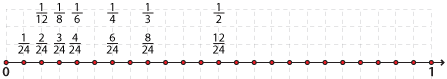# Year 7

## Number and Algebra

### Equivalent fractions and the use of the number line

Equivalent fractions are equal even though they have different numerators and denominators. Equivalent fractions mark the same place on a number line.

We can divide the interval [0, 1] on the number line into any number of equal intervals.

Consider the number 24. We can divide the interval [0, 1] into 24 equal intervals, each of length $$\dfrac{1}{24}$$. We can use these intervals to form:

• Two equal intervals in [0, 1], each of length $$\dfrac{1}{2}$$
• Three equal intervals in [0, 1], each of length $$\dfrac{1}{3}$$
• Four equal intervals in [0, 1], each of length $$\dfrac{1}{4}$$
• Six equal intervals in [0, 1], each of length $$\dfrac{1}{6}$$
• Eight equal intervals in [0, 1], each of length $$\dfrac{1}{8}$$
• Twelve equal intervals in [0, 1], each of length $$\dfrac{1}{12}$$.

This is illustrated on the number line belowDetailed description

The number 24 is useful because it has so many factors. The number 60 is even more useful. It is the smallest number with divisors of one, two, three, four and five, hence has many factors and there is some research which suggests that this is why it was used by the Babylonians as the base for their number system.

By writing fractions with the same denominator, that is, by finding an equivalent fraction, we can place all fractions in order on the number line.